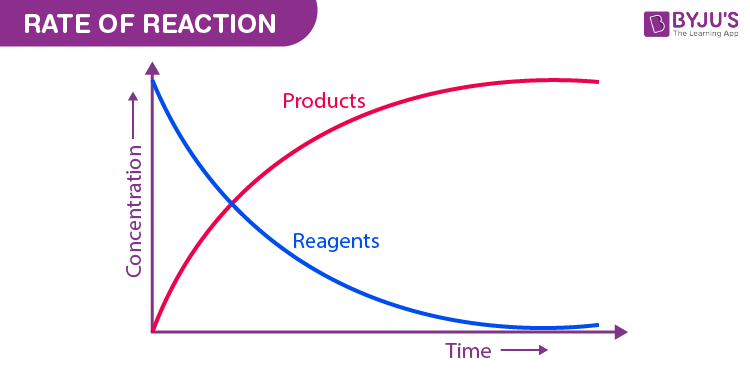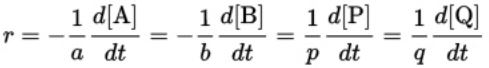# Rate Of Reaction

The rate of reaction refers to the speed at which the products are formed from the reactants in a chemical reaction. It gives some insight into the time frame under which a reaction can be completed. For example, the reaction rate of the combustion of cellulose in fire is very high and the reaction is completed in less than a second.

## What is Reaction Rate?

The rate of reaction or reaction rate is the speed at which reactants are converted into products. When we talk about chemical reactions, it is a given fact that rate at which they occur varies by a great deal. Some chemical reactions are nearly instantaneous, while others usually take some time to reach the final equilibrium.

This article aims to help students learn about and understand what exactly is the rate of reaction for a given chemical compound.As per the general definition, the speed with which a reaction takes place is referred to as the rate of a reaction.

For example, wood combustion has a high reaction rate since the process is fast and rusting of iron has a low reaction rate as the process is slow.

## Factors Affecting the Rate of Reaction

The various factions that can affect the rate of a chemical reaction are listed in this subsection.

#### Nature of the reaction

• The rate of reaction highly depends on the type and nature of the reaction. As mentioned earlier, few reactions are naturally faster than others while some reactions are very slow.
• The physical state of reactants, number of reactants, complexity of reaction and other factors highly influence the reaction rate as well.
• The rate of reaction is generally slower in liquids when compared to gases and slower in solids when compared to liquids. Size of the reactant also matters a lot. The smaller the size of reactant, the faster the reaction.

#### Effect of concentration on reaction rate

• According to the collision theory, the rate of reaction increases with the increase in the concentration of the reactants.
• As per the law of mass action, the chemical reaction rate is directly proportional to the concentration of reactants.
• This implies that the chemical reaction rate increases with the increase in concentration and decreases with the decrease in the concentration of reactants.
• Time plays a major role in changing the concentration of reactants and products. Therefore, even time is a vital factor affecting the reaction rate.

### Recommended Videos#### Pressure factor

• Pressure increases the concentration of gases which in turn results in the increase of the rate of reaction. The reaction rate increases in the direction of less gaseous molecules and decreases in the reverse direction.
• Thus, it can be understood that pressure and concentration are interlinked and that they both affect the rate of reaction.

#### How does temperature affect the reaction rate?

• According to collision theory, a chemical reaction that takes place at a higher temperature generates more energy than a reaction at a lower temperature.
• This is because colliding particles will have the required activation energy at high temperature and more successful collisions will take place.
• There are some reactions that are independent of temperature. Reactions without an activation barrier are examples of chemical reactions that are independent of temperature.

#### Solvent

The rate of reaction also depends on the type of solvent. Properties of solvent and ionic strength highly affect the reaction rate.

#### Order

The order of reaction manages how the reactant pressure or concentration affects the rate of reaction.

Electromagnetic radiation is a form of energy and its presence at the chemical reaction may increase the rate of reaction as it gives the particles of reactants more energy.

#### Intensity of Light

Even the intensity of light affects the rate of reaction. Particles absorb more energy with the increase in the intensity of light thereby increasing the rate of reaction.

#### Presence of Catalyst

• A catalyst can be defined as a substance that increases the rate of the reaction without actually participating in the reaction. The definition itself describes its effect on chemical reactions.
• The presence of a catalyst increases the speed of reaction in both forward and reverse reaction by providing an alternate pathway which has lower activation energy.

#### Surface Area of the Reactants

The surface area of reactants affects the rate of reaction. If the size of a particle is small, the surface area will be more and this increases the speed of heterogeneous chemical reactions.

## Rate of Reaction Formula

Let’s take a traditional chemical reaction.

a A + b B → p P + q Q

Capital letters (A&B) denote reactants and the (P&Q) denote products, while small letters (a,b,p,q) denote Stoichiometric coefficients.

As per IUPAC’s Gold Book, the rate of reaction r occurring in a closed system without the formation of reaction intermediates under isochoric conditions is defined as:Here, the negative sign is used to indicate the decreasing concentration of the reactant.

### Average Rate of reaction

Now let us consider the following reaction to understand even more clearly.

A → B

In this reaction a reactant A undergoes a chemical reaction to give a product B. It is a general convention to represent the concentration of any reactant or product as [reactant] or [product]. So the concentration of A can be represented as [A] and that of B as [B]. Let the time at which the reaction begins be the start time, that is t=0.

Let’s consider the following situation:

At t=t1,

The concentration of A=[A]1

The Concentration of B=[B]1

At t=t2,

The concentration of A=[A]2

The concentration of B=[B]2

Now we want to know the rate at which A (reactant) is disappearing and the rate at which the product B is appearing in the time interval between t1 and t2. Therefore,

The rate of Disappearance of A

$$\begin{array}{l}= \frac{[A]_{2} – [A]_{1}]}{t_{2} – t_{1}} = – \frac{\Delta [A]}{\Delta t}\end{array}$$

The negative sign shows that the concentration of A is decreasing.

Similarly,

Rate of disappearance of B

$$\begin{array}{l}= \frac{[B]_{2} – [B]_{1}]}{t_{2} – t_{1}} = \frac{\Delta [B]}{\Delta t}\end{array}$$

Since A is the only reactant involved in the reaction and B is the only product that is formed and as mass is conserved, the amount of A disappeared in the time interval Δt will be same as the amount of B formed during the same time interval. So we can say that

The rate of reaction = – Rate of disappearance of A = Rate of appearance of B

Therefore, the Rate of Reaction

$$\begin{array}{l}= – \frac{\Delta [A]}{\Delta t} = \frac{\Delta [B]}{\Delta t}\end{array}$$

The above terms for the rate of disappearance of A and rate of appearance of B are average rates of reaction. These rates give the rate of reaction for the entire time interval Δt and hence are called average rates of reaction.

## Instantaneous Rate of Reaction

What if we want to know the rate at which the reaction discussed above is proceeding at any instant of time and not for a given period of time? The average reaction rate remains constant for a given time period so it can certainly not give any idea about the rate of reaction at a particular instant.

This is where the instantaneous rate of reaction comes into the picture. Instantaneous rate of reaction is the rate at which the reaction is proceeding at any given time.

Suppose the value of the term Δt is very small and tends to zero. Now, we have an infinitesimally small Δt which is a very small time period and can be considered a particular instant of time. The average reaction rate will be the instantaneous rate of reaction.

Mathematically,

$$\begin{array}{l}Average\ Rate\ of\ Reaction = – \frac{\Delta [A]}{\Delta t} = \frac{\Delta [B]}{\Delta t}\end{array}$$

When Δt →0

$$\begin{array}{l}Instantaneous Rate of Reaction = – \frac{\Delta [A]}{dt} = \frac{\Delta [B]}{dt}\end{array}$$
$$\begin{array}{l}Instantaneous Rate of Reaction = – \frac{d[A]}{dt} = \frac{d[B]}{dt}\end{array}$$

The unit of rate of reaction is given by concentration/time that is (mol/L)/sec.

Meanwhile, chemical kinetics has gained a critically significant role in the world today. The reaction rate (both average and instantaneous) is enabling engineers and scientists around the globe to optimize the process parameters in order to get the most desired results from a chemical reaction in the most economical and safe way.

Chemical kinetics along with its critical role in the manufacturing industry has also served as a base for further advances in the fields of reaction engineering and biochemical engineering.

Any chemical reaction contains the following two constituents

• Reactants
• Products

The role these constituents play in chemical reactions is briefly described below. Important concepts in chemical reactions such as activation energy are also described.

#### Reactants

Substances which undergo chemical reactions are called reactants. In a chemical reaction, these reactants are converted into new substances.

#### Products

The substances which are the end products of a chemical reaction are called products. In other words, new substances that are formed due to the chemical reactions are all called products.

#### Activation Energy

Activation energy can be defined as the minimum amount of energy that is required to activate molecules or atoms so that they can undergo chemical transformation. This minimum energy is to overcome the energy barrier is called activation energy.

Similarly, chemical kinetics is a part of physical chemistry that is related to the study of reaction rates. It has many applications that include enzymology, chemical engineering, and environmental engineering.

In a chemical reaction, products are formed due to the collision between the reactant molecules.

The conditions for the collisions to form products are:

• Collisions should be effective.
• The right orientation of reactant molecules towards each other.
• All molecules should possess a minimum amount of energy to form product molecules.

As the chemical reaction advances, the concentration of reactants will decrease and the concentration of products will increase.